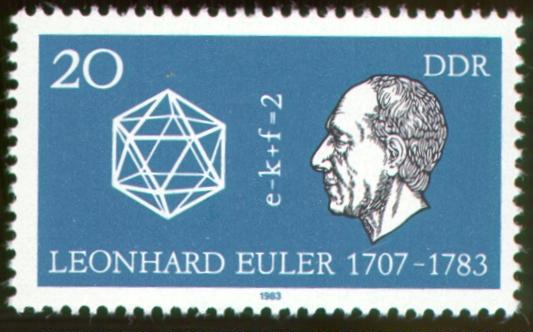Manfred BörgensMathematics on stamps stamp listnext stamp main pagedeutsche VersionGerman Democratic Republic 1983   Scott 2371

Leonhard Euler (1707 - 1783), icosahedron, polyhedral formula

Leonhard Euler is undoubtedly one of the most important mathematicians of all times. This page does not intend to give his biography - it would be too short (in relation to Euler's mathematical impact) or too long (in relation to the usual size of my mathematical stamps pages). The internet provides some good biographies; an outstanding description of Euler's life in Basel, Berlin and St Petersburg and of his mathematical achievements can be found in the MacTutor History of Mathematics archive of the Scottish university St Andrews.

The stamp honoured Euler on the occasion of the 200th anniversary of his death and shows an icosahedron, one of the five Platonic solids. A Platonic solid is a convex polyhedron whose faces are congruent regular polygons and at whose vertices meet the same number of faces. The other Platonic solids are tetrahedron, cube, octahedron and dodecahedron. The faces of an icosahedron are 20 congruent regular triangles. In some respects it is the biggest Platonic solid: It has the highest number of faces (20), of edges (30, sharing this record with the dodecahedron), and at its vertices meet the maximally possible 5 faces. There is an interesting connection between the icosahedron and the soccer ball: Cut all vertices of an icosahedron; this will result in 12 additional faces (pentagons, often in black colour on the soccer ball); the original 20 triangles become hexagons (often in white colour on the soccer ball).

The stamp also displays Euler's polyhedral formula:

e - k + f = 2

e  stands for the number of vertices (German "Ecken")k  for the number of edges (German "Kanten") and  f  for the number of faces (German "Flächen"). This formula holds for all convex polyhedra. For the icosahedron the formula gives:

12 - 30 + 20 = 2

For the soccer ball the formula gives:

60 - 90 + 32 = 2

In 1988, mathematicians worldwide were asked to vote for their favourite theorems. Euler's polyhedral formula finished second, Euclid's theorem "There are exactly five Platonic solids" finished fourth (see David Wells, Which is the most beautiful?, Mathematical Intelligencer, 12 (1988), 37-41).

More Euler stamps can be viewed here. A Swiss banknote also shows Euler's portrait.

last update  2004-10-10
stamp list   |    next stamp
Manfred Börgens   |    main page